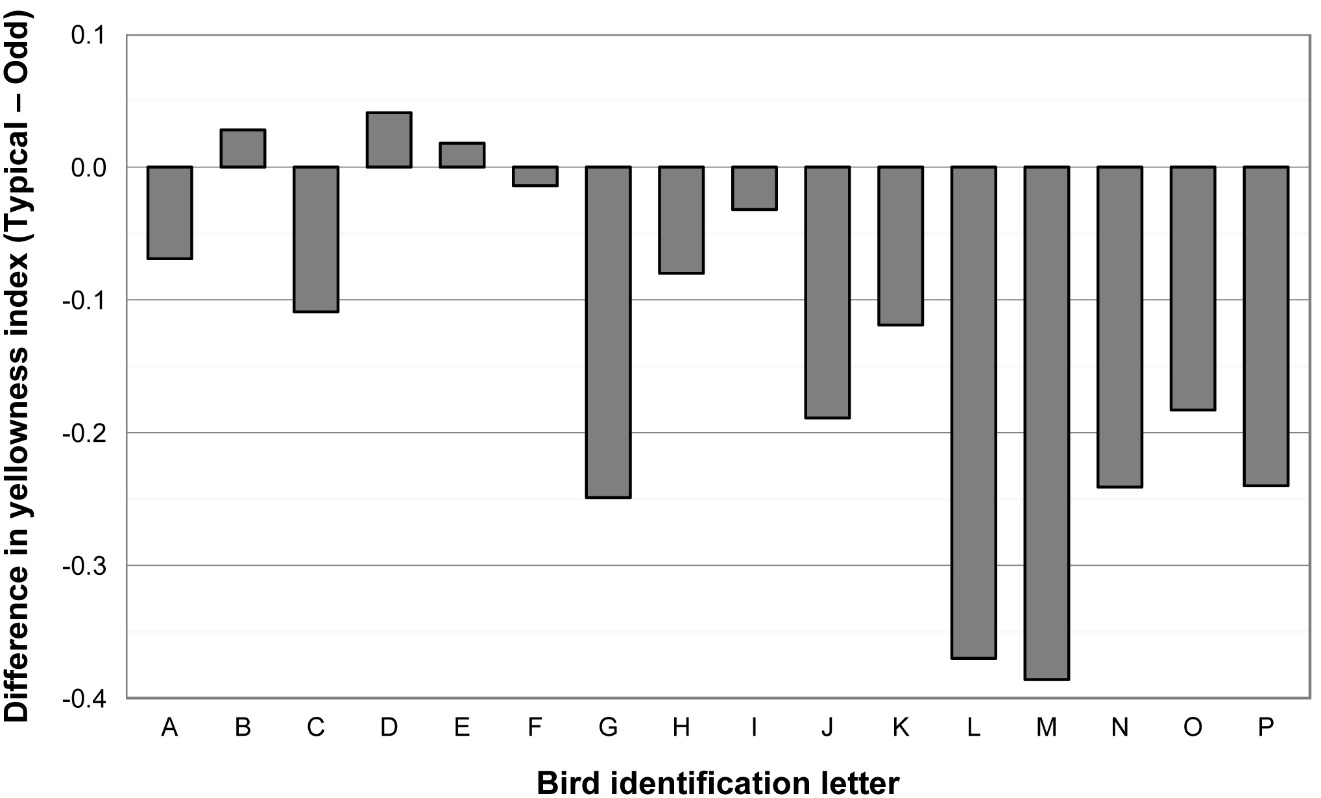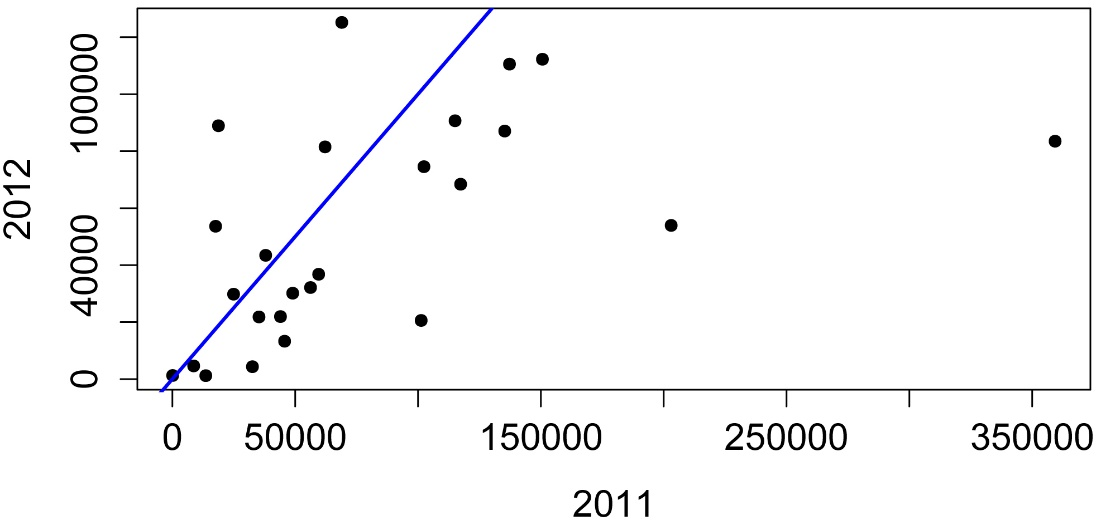## An R Companion for the Handbook of Biological Statistics

Salvatore S. Mangiafico

# Paired t–test

Paired t-tests can be conducted with the t.test function in the native stats package using the paired=TRUE option.  Data can be in long format or short format.  Examples of each are shown in this chapter.

As a non-parametric alternative to paired t-tests, a permutation test can be used.  An example is shown in the “Permutation test for dependent samples” section of this chapter.

### Examples in Summary and Analysis of Extension Program Evaluation

SAEEPER: One-way Permutation Test of Symmetry for Paired Ordinal Data

### Packages used in this chapter

The following commands will install these packages if they are not already installed:

if(!require(ggplot2)){install.packages("ggplot2")}
if(!require(coin)){install.packages("coin")}
if(!require(pwr)){install.packages("pwr")}

When to use it

The horseshoe crab example is shown at the end of the “How to do the test” section.

Null hypothesis

Assumption

How the test works

See the Handbook for information on these topics.

Examples

The flicker feather example is shown in the “How to do the test” section.

Graphing the results

Plots are shown in the “How to do the test” section.

### How to do the test

#### Paired t-test, data in wide format, flicker feather example

### --------------------------------------------------------------
### Paired t-test, Flicker feather example, p. 185
### --------------------------------------------------------------

Input = ("
Bird   Typical  Odd
A     -0.255   -0.324
B     -0.213   -0.185
C     -0.190   -0.299
D     -0.185   -0.144
E     -0.045   -0.027
F     -0.025   -0.039
G     -0.015   -0.264
H      0.003   -0.077
I      0.015   -0.017
J      0.020   -0.169
K      0.023   -0.096
L      0.040   -0.330
M      0.040   -0.346
N      0.050   -0.191
O      0.055   -0.128
P      0.058   -0.182
")

##### Paired t-test

t.test(Data\$Typical,
Data\$Odd,
paired=TRUE,
conf.level=0.95)

t = 4.0647, df = 15, p-value = 0.001017

mean of the differences

0.137125

##### Simple plot of differences

Difference = Data\$Odd - Data\$Typical

plot(Difference,
pch = 16,
ylab="Difference (Odd – Typical)")

abline(0,0, col="blue", lwd=2)A simple plot of differences between one sample and the other.  Points below the blue line indicate observations where Typical is greater than Odd, that is where (OddTypical) is negative.

##### Simple 1-to-1 plot of values

plot(Data\$Typical, Data\$Odd,
pch = 16,
xlab="Typical feathers",
ylab="Odd feathers")

abline(0,1, col="blue", lwd=2)Plot of paired samples from a paired t-test.  Circles below or to the right of the blue one-to-one line indicate observations with a higher value for Typical than for Odd.

##### Checking assumptions of the model

Difference = Data\$Odd - Data\$Typical

hist(Difference,
col="gray",
main="Histogram of differences",
xlab="Difference")Histogram of differences of two populations from a paired t-test.  Distribution of differences should be approximately normal.  Bins with negative values indicate observations with a higher value for Typical than for Odd.

##### Graphing the results

Data\$Difference = Data\$Odd - Data\$Typical

library(ggplot2)

ggplot(Data,
aes(x = Bird,
y = Difference)) +
geom_bar(stat = "identity",
fill = "grey50",
colour = "black",
width = 0.6) +
scale_y_continuous(breaks = seq(-0.4, 0.1, 0.1),
limits = c(-0.4, 0.1),
expand = c(0, 0)) +
#ggtitle("Chart title") +
labs(x = "Bird identification letter",
y = "Difference in yellowness index (Typical – Odd)")  +
theme_bw() +
theme(panel.grid.major.x = element_blank(),
panel.grid.major.y = element_line(colour = "grey50"),
plot.title = element_text(size = rel(1.5),
face = "bold", vjust = 1.5),
axis.ticks.x = element_blank(),
axis.ticks.y = element_blank(),
axis.title.y = element_text(face = "bold",
vjust= 1.8),
axis.title.x = element_text(face = "bold",
vjust= -0.8)
)

#     #     ##### Paired t-test, data in wide format, horseshoe crab example

### --------------------------------------------------------------
### Paired t-test, Horseshoe crab example, pp. 181–182
### --------------------------------------------------------------

# Note, if you use "2011" as a variable name,
#   the read.table function will convert it to "X2011"

Input = ("
Beach               Year.2011    Year.2012
'Bennetts Pier'      35282        21814
'Big Stone'         359350        83500
'Cape Henlopen'      49005        30150
'Fortescue'          68978       125190
'Fowler'              8700         4620
'Gandys'             18780        88926
'Higbees'            13622         1205
'Highs'              24936        29800
'Kimbles'            17620        53640
'Kitts Hummock'     117360        68400
'Norburys Landing'  102425        74552
'North Bowers'       59566        36790
'North Cape May'     32610         4350
'Pickering'         137250       110550
'Pierces Point'      38003        43435
'Primehook'         101300        20580
'Reeds'              62179        81503
'Slaughter'         203070        53940
'South Bowers'      135309        87055
'South CSL'         150656       112266
'Ted Harvey'        115090        90670
'Townbank'           44022        21942
'Villas'             56260        32140
'Woodland'             125         1260
")

##### Paired t-test

t.test(Data\$Year.2011, Data\$Year.2012,
paired=TRUE,
conf.level=0.95)

t = 2.1119, df = 24, p-value = 0.04529

mean of the differences

28225.4

##### Simple 1-to-1 plot of values

plot(Data\$Year.2011, Data\$Year.2012,
pch = 16,
xlab="2011",
ylab="2012")

abline(0,1, col="blue", lwd=2)Plot of paired samples from a paired t-test.  Circles below and to the right of the blue one-to-one line indicate observations with a higher value for 2011 than for 2012.

Difference = Data\$Year.2012 - Data\$Year.2011

hist(Difference,
col="gray",
main="Histogram of differences",
xlab="Difference")Histogram of differences in two populations from paired t-test.  Distribution of differences should be approximately normal.  Bins with negative values indicate observations with a higher score for 2011 than for 2012.

#     #     #

#### Paired t-test, data in long format

### --------------------------------------------------------------
### Paired t-test, long format data, Flicker feather example, p. 185
### --------------------------------------------------------------

Input = ("
Bird    Feather   Length
A       Typical   -0.255
B       Typical   -0.213
C       Typical   -0.19
D       Typical   -0.185
E       Typical   -0.045
F       Typical   -0.025
G       Typical   -0.015
H       Typical    0.003
I       Typical    0.015
J       Typical    0.02
K       Typical    0.023
L       Typical    0.04
M       Typical    0.04
N       Typical    0.05
O       Typical    0.055
P       Typical    0.058
A       Odd       -0.324
B       Odd       -0.185
C       Odd       -0.299
D       Odd       -0.144
E       Odd       -0.027
F       Odd       -0.039
G       Odd       -0.264
H       Odd       -0.077
I       Odd       -0.017
J       Odd       -0.169
K       Odd       -0.096
L       Odd       -0.33
M       Odd       -0.346
N       Odd       -0.191
O       Odd       -0.128
P       Odd       -0.182
")

### Note: data must be ordered so that the first observation of Group 1
###   is the same subject as the first observation of Group 2

t.test(Length ~ Feather,
data=Data,
paired=TRUE,
conf.level=0.95)

t = -4.0647, df = 15, p-value = 0.001017

mean of the differences

-0.137125

#     #     #

#### Permutation test for dependent samples

This permutation test is analogous to a paired t-test.

### --------------------------------------------------------------
### Paired two-sample permutation test, long format data
### Flicker feather example, p. 185
### --------------------------------------------------------------

Input = ("
Bird    Feather   Length
A       Typical   -0.255
B       Typical   -0.213
C       Typical   -0.19
D       Typical   -0.185
E       Typical   -0.045
F       Typical   -0.025
G       Typical   -0.015
H       Typical    0.003
I       Typical    0.015
J       Typical    0.02
K       Typical    0.023
L       Typical    0.04
M       Typical    0.04
N       Typical    0.05
O       Typical    0.055
P       Typical    0.058
A       Odd       -0.324
B       Odd       -0.185
C       Odd       -0.299
D       Odd       -0.144
E       Odd       -0.027
F       Odd       -0.039
G       Odd       -0.264
H       Odd       -0.077
I       Odd       -0.017
J       Odd       -0.169
K       Odd       -0.096
L       Odd       -0.33
M       Odd       -0.346
N       Odd       -0.191
O       Odd       -0.128
P       Odd       -0.182
")

library(coin)

independence_test(Length ~ Feather | Bird,
data = Data)

Asymptotic General Independence Test

Z = -2.8959, p-value = 0.003781

#     #     #

### Power analysis

#### Power analysis for paired t-test

### --------------------------------------------------------------
### Power analysis, paired t-test, pp. 185–186
### --------------------------------------------------------------

Detect  =  0.1                    # Difference in means to detect
SD      = 0.135                   # Standard deviation of differences

Cohen.d = Detect/SD

library(pwr)

pwr.t.test(
n = NULL,                   # Number of _pairs_ of observations
d = Cohen.d,
sig.level = 0.05,           # Type I probability
power = 0.90,               # 1 minus Type II probability
type = "paired",            # paired t-test
alternative = "two.sided")

Paired t test power calculation

n = 21.16434

NOTE: n is number of *pairs*

#     #     #